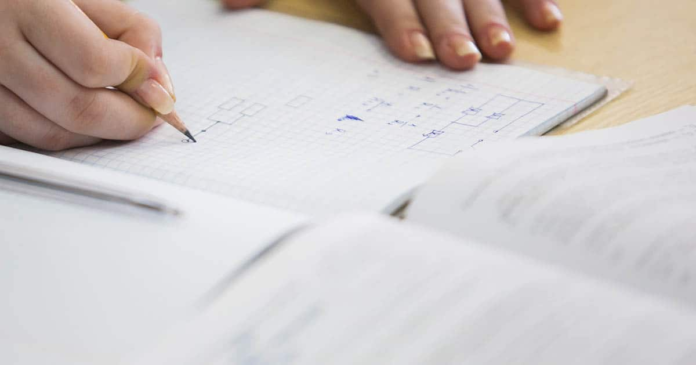# Tips and Tricks to Prepare Effectively for your Math Exam

0
1368In academic life, Mathematics is a compulsory as well as an important subject. In early education, kids should be introduced to the concepts of Mathematics. It is helpful for kids to get familiarized with the basic terms and concepts of Math at an early age. Enhancing their Math at the earliest will make elementary education a little convenient. At the age of three years old, kids should be introduced to Math concepts. Math is a subject that is purely based on practice. Most students are scared of Math as they do not have a basic understanding of the subject. However, if one studies Mathematics properly, then improving their skills would be a cakewalk. All students need to do is devote time for the subject regularly and work on building the concepts. Here we have provided some techniques which will help students in preparing for the Math exam.

Most of the students or kids find the subject of Maths boring, complex, tough and difficult. But, it is a subject that cannot be avoided at any stage. For kids, to make it more interesting and fun-loving parents can take the help of games like Math games for grade 2, etc. Students can be well-versed with Maths by involving themselves in constant practice. They should be thorough with the concepts, formulas, equations, theorems, etc. to make the subject interesting and easier to understand.

## 5 Tips for Solving Math Problems

### 1) Understanding Rather than Memorising Math

Students should focus on understanding the basics of Mathematics. Instead of memorising the steps to solve the questions, they should focus on the approach of solving it. It is therefore imperative for students to diligently solve the exercise questions from the textbook to gain practice. If students find themselves stuck somewhere then they can refer to their respective Maths textbook. So, don’t mug up Math just for the sake of scoring marks in the exam. Instead, focus on building the concepts.

### 2) Start By Solving the Examples

Each topic is explained with solved examples in the prescribed Math Book. So, before solving the exercise problems, students must go through the examples and try to grasp the concepts. Following which, they should make note of their shortcomings and work on them to improve their understanding related to those topics. This would give them a different approach to solving the questions.

### 3) Note Down All the Crucial Concepts

Early Math consists of numbers, multiplication, addition, subtraction, etc. So, students should make a proper note of the concepts related to the mentioned topics like chart of multiplication tables, division method, subtraction method, etc. So, it is advised that they make a separate notebook where chapter wise concepts and topics are noted down. These notes will help them in quick revision during exams.

### 4) Clear All Doubts

It’s normal to have doubts when students are solving Math problems. But do not let doubts persist for long periods. Students must get them cleared as soon as possible either by discussing them with friends or with the teacher. If there is any doubt regarding any concept they can take help of their respective Maths textbook.

### 5) Practice Math Daily

The best way to master Math is to solve the sums. So, students must devote at least 1 hour to the subject in their daily study schedule. This would lead to a gradual improvement in the performance of students and would also consequently enhance their interest in Math.

Learning Maths through games makes the subject more exciting and fun-loving. It is a great way to enhance your kids’ learning. Kids can get a variety of games related to Maths in the market as well as on the web. Play the games and make your mathematical skills polished and perfect. In the market, parents can find games based on Maths for different age groups and grades like Math games for grade 3, grade 4, etc.